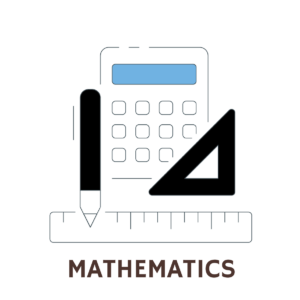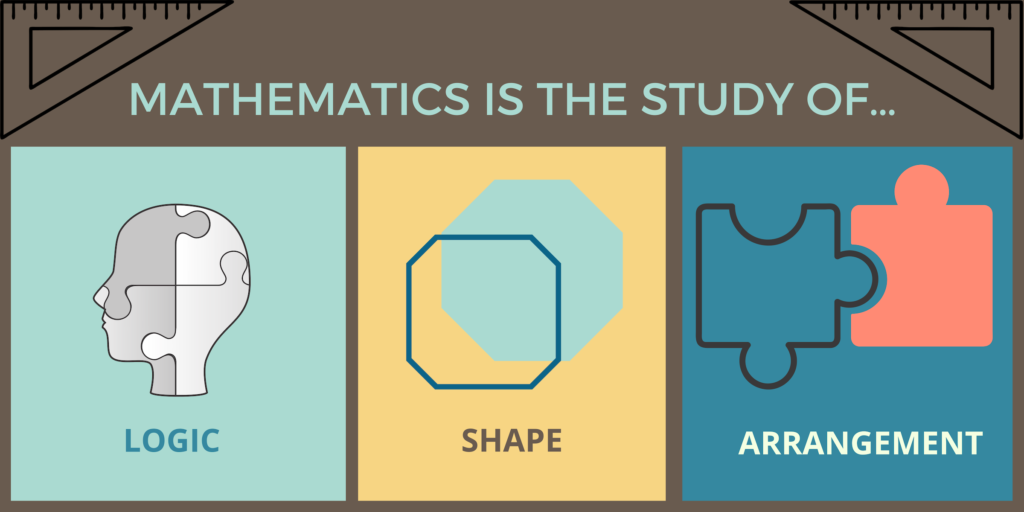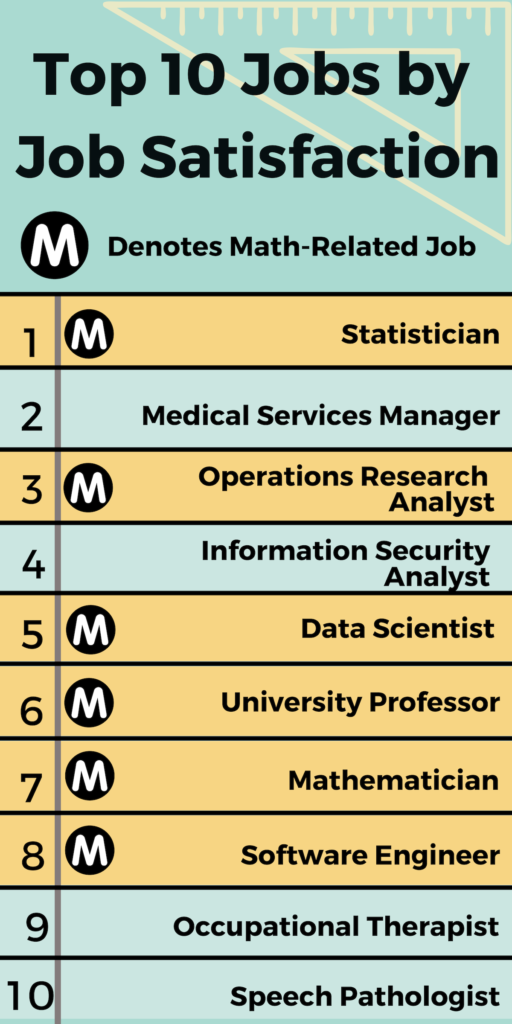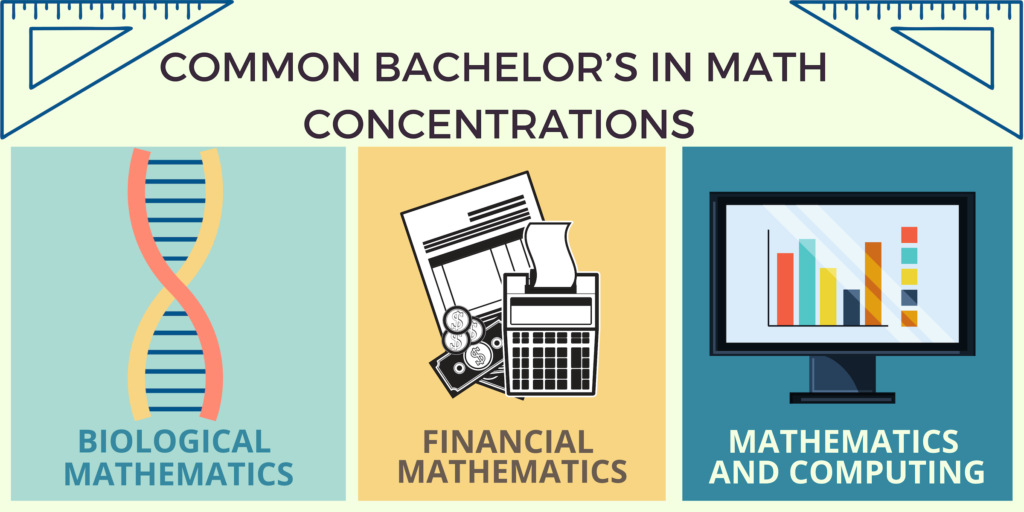• FIND A DEGREEIf you’re already into math then you likely already know that mathematics is a language. And much as languages that are spoken, the language of numbers can be applied in my different ways.

Like any other language, you must first learn the basic rules. From kindergarten through the 12th grade, the grammar and syntax of numbers fill math classes. This is where many lose interest in math. But not you. If you’re interested in pursuing a degree in mathematics at the undergraduate or graduate levels, you want to learn how to apply math to solve really interesting problems.The good news is that the undergraduate years are where you really start to learn how to solve complex, real-world problems with math. From fine-tuning the most complex algorithms to verifying that a statistical approach is correct for a study, mathematics is at the center of many university-based and real-world disciplines. Plus, universities are home to faculty that can help you dive deeper into the theoretical problems in math.

Whatever your choice, for those serious about enhancing their mathematical understanding, higher education is a must.

Here at DegreeQuery we cover all types of degree programs in higher ed. And mathematics is no exception. Read more to find out what exactly you can do with a degree in mathematics!

Mathematics Degree FAQs

### What is the study of Mathematics?Before we jump into what you can do with a degree in mathematics, let’s examine what the study of mathematics is.

At its most general level, mathematics is the seeking and using of patterns in numbers. Many times these patterns mimic or are helpful in the representation of some phenomena out in the world. When this occurs, new branches of applied mathematics crop up.

So when we are talking about mathematics, we’re both talking about theoretical mathematicians that seek patterns as well as those who use mathematics for useful applications. Careers that work in applied mathematics include the following:

• Mathematicians
• Statisticians
• Actuaries
• Accountants
• Financial Analysts
• Data Scientists
• Natural Scientists
• Social Scientists
• Economists
• Computer Scientists
• Educators
• And many others

.

At an undergraduate level, courses in mathematics may include the following subjects:

• Calculus I-III
• Functions and Modeling
• Math for Engineering
• Differential Equations
• Discrete Math
• Statistics
• Financial Math
• Mathematical Biology
• Multiple Courses on Analysis
• Differential Geometry
• Numbers Theory
• History of Mathematics
• And More

In graduate settings or on-the-job applications, mathematicians and related jobs will often focus primarily on a handful of areas. Below are the major areas one can focus on in the study of and application of mathematics.
Areas of Pure Math

• Foundations
• Arithmetic
• Algebra
• Analysis
• Combinatorics
• Geometry and topology

Areas of Applied Math

• Probability and statistics
• Computational Sciences
• Numerical Analysis
• Computer Algebra
• Physical sciences
• Mechanics
• Mechanics of Structures
• Mechanics of Deformable Solids
• Fluid Mechanics
• Particle Mechanics
• Operations Research
• Mathematical Programming

### What Fields of Study Heavily Utilize Mathematics?

The first step to answering “what you can do” with a mathematics degree is to look at what fields heavily utilize mathematics. After a discussion of these fields, we will look at what degree options can help you to get to your desired role or focus.Science, Technology, Engineering, and Math (STEM) disciplines are in high demand across many industries, and while there aren’t a high degree of jobs that are 100% math, job candidates with strong quantitative skills are truly prized.

Some of the most common job roles relying heavily on include the following:

• Mathematicians and Statisticians work with formulas and data to help solve important problems in government, academic, and industry settings. They are in high demand across health care, engineering, business, and computing fields.
• Actuaries analyze — in financial terms — uncertainty. Commonly employed in finance and insurance settings, actuaries use financial theory, statistics, and other mathematical modeling tools to explore and explain the risks of certain events happening.
• Data Scientists apply statistics, data analysis, and machine learning to create insight from data. Of other STEM fields, data scientists are most closely related to statisticians that rely on a purely digital set of tools and data.
• Natural Scientists apply a range of mathematical methods depending on their field of focus. Statistics and probability are regularly employed in scientific disciplines. A range of applied science disciplines were developed in tandem with natural scientists including mechanics, fluid mechanics, and particle mechanics. These disciplines are integrally tied to both engineering and physics.
• Engineers employ mathematics in the form of statistics, probability, and the study of mechanics of structures, fluids, and particles. Besides mathematics and physics, engineering is often viewed as the most “math heavy” discipline taught at universities.
• Cryptographers have long been employed in a range of settings, from the famous Enigma machine of WWII to ancient codes for communication between Roman Armies. Today cryptographers are most heavily employed in cyber security and information assurance related disciplines. Cryptography most heavily utilizes abstract geometry and number theory.

### How Much Do Math Majors Make?

Math majors are one of the most sought-after majors in a variety of fields. Additionally, math majors score the highest of any other major on standardized tests for graduate school (see our section on how mathematics prepares you for grad school below).The salary of math majors depends on a number of factors, but what we can look at are the average salaries for common jobs that math majors hold after graduation.

Of the four careers most closely related to mathematics, average salaries are high:
Average Salaries for Math-Related Careers

• Mathematician: \$103,010
• Actuaries: \$101,560
• Statisticians: \$84,760
• Operations Research Analysts: \$81,390

There are of course many careers that mathematics majors or graduate degree recipients can enter into. Of roles that prize mathematicians with a little additional training, here are common salaries:
Average Salaries in Careers Mathematicians Can Work In

• Physicists: \$117,220
• Computer and Information Research Scientists: \$114,520
• Financial Analysts: \$84,300
• High School Math Teachers: \$59,170
• Data Scientist: \$118,709
• Cryptographer: \$112,560
• University Math Instructor: \$74,460

Additional concentrations in mathematics that are in high demand in the workforce include graduates that have focused on biological mathematics, financial mathematics, and the teaching of mathematics.

### How Mathematics Prepares You For Grad SchoolQuantitative thinking — as is taught in mathematics degrees — is prized in a wide variety of fields. It’s also a crucial skill for excelling at standardized tests for graduate schools. Mathematics majors score higher — on average — than any other major on both LSAT and GMAT tests. These are the predominant tests for entry into graduate programs in law school as well as business.

The bulleted list below notes how many points above or below the average GMAT and LSAT scores individual majors perform.

Top Three Majors for LSAT Scores And Difference From Average Score

• Mathematics: +12.8%
• Economics: +9.6%
• Philosophy: +8.7%

Bottom Three Majors for LSAT Scores And Difference From Average Score

• Education: -8.7%
• Political Science: -1.6%

Top Three Majors for GMAT Scores and Difference From Average Score

• Mathematics: +13.3%
• Philosophy: +11%
• Chemistry: +7.5%

Bottom Three Majors for GMAT Scores and Difference From Average Score

• Sociology: -5%
• Education: -4.2%

Mathematical techniques can be applied to many disciplines, including those that just need a strong basis in problem-solving in logic. This makes math a great undergraduate major to take into graduate school in another discipline.

Some common graduate programs pursued by mathematics majors include:

• Mathematics
• Statistics
• Accounting
• Finance
• Computer Science
• Engineering
• Physics
• Law
• Education

For some context on just how well a mathematics degree prepares you for graduate schools of a variety of types, let’s dive into average GMAT scores by major.

GMAT tests are graded on a scale between 200 and 800, with an average score of around 500. The average mathematics major taking the GMAT scores 13.3% higher than the average. This means that the average mathematics major taking the GMAT scores around 580, 80 points above average.

Keep in mind there are many parts of a graduate business school application. Standardized tests like the GMAT, however, are generally one of the largest components.

Many top-tier business schools — currently ranked between 60th and 100th in the nation — have average GMAT score of slightly over 600. These averages are the middle of what is generally a 20 points spread in either direction. This means that the GMAT score of mathematics majors is high enough to gain admittance into many top 100 graduate business programs. Furthermore, besides philosophy or economics — the only other two majors to boast scores close to as high on the GMAT — it’s the only major that can boast such high results.

### What is the Career Outlook for Those With Math Degrees?

If we haven’t made it clear already, mathematics majors degree holders are in high demand in many disciplines. From high school education to “quants” in high finance, mathematicians provide the “secret sauce” to many organization’s success. There also aren’t many mathematicians. This makes those with math degrees even more desirable.Below we’re going to look at the career outlook for those with math degrees through two lenses: mathematics graduates that work as mathematicians or statisticians, and those that work in more applied fields, or in fields related to mathematics.

Career outlook for mathematicians and statisticians
In 2016, there were slightly over 40,000 workers in roles title mathematician or statistician in America. From 2016-2026, an estimated 13,500 more jobs in these fields are expected to be available. So in the span of a decade, mathematician and statistician jobs will have increased in number by a third (33%).

The average salary for these roles as of 2016 is \$84,760. With the largest employment settings of those with jobs titled mathematician or statistician in the following locations:
Locations of Work for Mathematicians

• Federal government
• Research and development in the physical sciences, engineering, and life sciences
• Colleges and Universities
• Finance and Insurance
• And Management, scientific, and technical consulting

Locations of Work for Statisticians

• Federal government
• Research and development in the physical sciences, engineering, and life sciences
• Insurance
• Healthcare and social assistance
• Colleges and Universities

Career outlook for Mathematics-Heavy Roles
Perhaps the largest mathematics related role by number of jobs is mathematics instructor. This is followed by a wide range of mathematics-related roles including actuary, data scientist, certain engineering roles, and operations research analysts. The career outlooks of these career types may be seen below:
Growth in Job Postings by 2026

• Actuary: +22%
• Data Scientists: +19%
• Electrical Engineers: +9%
• Operations Research Analysts:+27%
• Math Educator: +12%

Salary of Mathematics-Heavy Roles

• Actuary: \$101,560
• Data Science: \$114,520
• Electrical Engineers: \$97,970
• Operations Research Analysts: \$81,390
• Math Educator: \$55,035

Interested in job interview tips? Check out DegreeQuery’s coverage of how to interview for some of the most common mathematics jobs below:

### Job Satisfaction for MathematiciansEarnings are only one component of whether you love you job (though an important one). A number of nuanced surveys have ranked job satisfaction for mathematicians and related jobs as some of the highest of any jobs. In a survey of 200 career types in 2017, CareerCast ranked a number of mathematics-related careers as some of the highest for job satisfaction of any. Some of the highlights include the following roles.

• Statistician: Ranked #1 for Job Satisfaction
• Operations Research Analyst: Ranked #3 for Job Satisfaction
• Data Scientist: Ranked #5 for Job Satisfaction
• University Professor: Ranked #6 for Job Satisfaction
• Mathematician: Ranked #7 for Job Satisfaction
• Software Engineer: Ranked #8 for Job Satisfaction

With 6 of the top 10 jobs rated for highest job satisfaction, it’s hard to go wrong with a mathematics degree!

### Types of Mathematics Degrees

Mathematics and related degrees are available at the associate’s through doctoral levels. You choice of what degree level to pursue will help to dictate what jobs you may qualify for, and rely on your current education level, the cost of schooling, and the time you can set aside to pursue further education. Below we’ll work through descriptions of common degree programs in mathematics.

### Associates Degree in Mathematics

Associate’s degrees in mathematics are typically transfer programs in which students transfer to a four-year program in accounting, mathematics, actuarial science, or engineering once they have completed their associate’s.

As with associate’s degrees in other disciplines, an associate’s in mathematics typically requires 60 credit hours of study which often requires 1.5-2 years of study. Students first endeavor upon general education requirements. General education requirements help to ensure that graduates are well-rounded and analytical thinkers. Courses needed for this portion of your study may include courses in the humanities, social sciences, the arts, hard sciences, and writing.

Along with completing general education courses, mathematics majors in associate’s programs will often complete courses in the following:

Sample Mathematics Curriculum At the Associate’s Level

• Precalculus
• Calculus I, II, and III
• Linear Algebra
• Discrete Mathematics
• Statistics
• Several Electives in Math

As you can see, mathematics at the associate’s level typically just gets you started on your higher education mathematics path. Bachelor’s degrees in mathematics, statistics, actuarial science, accounting, or engineering pursue mathematics in much greater detail.

### Bachelor’s Degrees in MathematicsA bachelor’s in math or related disciplines is the standard degree for entry-level positions in engineering, accounting, actuarial science, statistics, or math. Bachelor’s degrees typically require around 120 semester credit hours to complete. These degrees typically require 4 years to complete, though completing a bachelor’s in 3-6 years is common without a prior associate’s degree.

As with associate’s degrees in math, general education requirements help to ensure mathematics majors are well-rounded and analytical thinkers. Courses in general education may include courses in the humanities, arts, social sciences, hard sciences, and writing. After completion of your general education requirements, you’ll move further into major requirements. We’ve detailed major requirements for math-heavy degrees below:

Curriculum for Bachelor’s in Mathematics Degree

• Probability and Statistics
• Pre-Calculus
• Calculus I, II, and III
• Real Analysis I and II
• Linear Algebra
• Abstract Algebra
• Differential Equations
• Geometry
• Stochastic Processes
• Mathematical Programming

Bachelor’s degrees in mathematics are typically offered in B.A. and B.S. format. Additionally, some schools may offer degrees in both mathematics and applied mathematics. Mathematics degrees push for depth of understanding, delving into more advanced pure mathematics. Applied mathematics degrees push students to understand applications of mathematics, including a greater focus on data science, statistics, analysis, and mathematics programming.

Some schools offer specializations in math at the undergraduate level. Common specializations include:

• Biological Mathematics
• Financial Mathematics
• Mathematics and Computing

Curriculum for Bachelor’s in Actuarial Science Degree
Actuarial science helps to prepare future actuaries. An actuary compiles and analyses statistics to help to assess risk. Actuaries commonly work for insurance companies, in financial settings, and for the government.

As we noted above in our career section, actuaries hold some of the highest job satisfaction rates of any career. And the most common degree for starting an actuarial career is a bachelor’s in actuarial science. A common curriculum for an actuarial science major may be seen below.

• Calculus I-V
• Intro to Actuarial Science
• Microeconomics
• Macroeconomics
• Linear Algebra
• Statistics and Probability for Actuarial Science
• Finance
• Differential Equations
• Mathematical Computing
• Graph Theory
• Linear Programming
• Electives

Curriculum for Bachelor’s in Statistics Degree
Statisticians boast some of the highest job satisfaction of any jobs. And the degree required for entry-level positions in statistician roles is a bachelor’s in mathematics or statistics. Below we’ve detailed a common curriculum for a statistics major at the bachelor’s level.

• Calculus I, II, and III
• Linear Algegra
• Statistics I and II
• Experimental Design
• Regression Analysis
• Computer Science I and II
• Data Management
• And electives

Degrees related to mathematics
A handful of other degrees involve a great deal of mathematics, and are often coupled as minors or double majors with a mathematics degree due to the overlap and valuable skills learned. Related degrees include:

• Physics
• Engineering
• Computer Science
• Accounting
• Finance

If you think you might be interested in a bachelor’s degree in mathematics, make sure to check out DegreeQuery’s ranking of the best bachelor’s in mathematics degree programs today!

If you’re wondering just how much math is present in some of the above degrees, make sure to check out our FAQs on the relationship between math and related subject:

### Master’s Degrees in Mathematics

For those looking to truly stand out in their field, master’s degrees in mathematics, statistics, and actuarial science are truly in high demand.

Master’s degrees in mathematics allow students to specialize more in one area of applied mathematics. For students looking to pursue research in pure mathematics, a doctoral degree is generally pursued after a bachelor’s degree. Students of doctoral programs are awarded a master’s degree part way through their course of study.

Common specializations in master’s level mathematics degrees include:

• General Mathematics
• Mathematics Education
• Computational Science and Engineering
• Computational Finance
• Computational Biology
• Statistics
• Actuarial Science

Master’s degrees generally require 1.5-2 years of study, and require between 30 and 54 credit hours for completion. Additionally, students at the master’s level are commonly required to create a capstone project or complete a master’s level thesis. A thesis is generally a hundred+ page survey of current literature within the field in which you are studying. Unlike a dissertation — which is original research — a master’s thesis shows that you are up-to-date on current research in your field and that you can think critically about issues in your field.

Common curricula for master’s-level math-related degrees are noted below. Though students may generally tailor their course of study more than at undergraduate levels.

Master’s in Mathematics Courses
Some selection of the following courses is common:

• Two graduate level analysis courses
• Discrete Math and Algebra
• Geometry and Topology
• Differential Equations
• Probability and Statistics
• Numerical Analysis
• A thesis

If you think you might be interested in a master’s in mathematics degree program, make sure to check out DegreeQuery’s coverage of the top programs for master’s in math today!
Master’s in Statistics Courses
Some selection of the following is common:

• Graduate Statistics I and II
• Design and Analysis of Experiments
• Modeling and Regression Analysis
• Analysis
• Statistical Estimation
• Testing Hypotheses
• Multivariate Stat Analysis
• Time-Series Analysis
• Stochastic Processes
• Bayesian Statistics
• Biological Statistics
• Financial Statistics
• Data Mining
• Reliability Theory
• Among others

Master’s in Actuarial Science Courses
Some selection of the following is common:

• Probability Theory
• Statistical Inference
• Theory of Interest
• Actuarial Methods
• Actuarial Models
• Finance and Economics Models
• Linear Regression and Time Series
• Bayesian Statistics
• Stochastic Processes
• Industry-Specific Courses

Master’s in Mathematics Education
One common route for bachelor’s in education holders to take is to pursue a teaching position in mathematics for primary or secondary school. Many master’s in mathematics programs help to lead to licensure in these subjects for the purposes of teaching in public schools. Below are some of the most common courses in this degree type:

• Calculus I and II
• Statistics I and II
• Linear Algebra
• Abstract Algebra
• Trigonometry
• Mathematics Teaching
• Teaching Algebra
• Teaching Geometry
• Teaching Calculus
• Teaching Statistics
• History of Math and Technology

### Doctoral Degrees in Mathematics

Doctorate of Philosophy (Ph.D.’s) in mathematics are the pinnacle of both applied and pure mathematics training. Ph.D.’s are research doctorates, meaning central goals of these programs are to prepare students to advance research in their field. As part of this goal, Ph.D. candidates in mathematics must submit a dissertation — or several-hundred-page publication of original research — to obtain a doctoral degree.

Doctoral programs in mathematics typically accept candidates with bachelor’s in mathematics, though some will allow entry for those with master’s degrees. Typically, doctoral candidates receive master’s degrees in mathematics part way through their doctoral course of study.

Doctoral programs in mathematics typically take 4-6 years to complete. Typical coursework includes the following, though a large portion of the program will also revolve around your original research:

• Analytic number theory
• Algebraic number theory
• Combinatorics
• Differentiable manifolds
• Partial differential equations
• Logic

Typically doctoral degrees allow students to specialize much more than other degree levels. Doctoral degrees in mathematics typically require students to choose between applied and pure mathematics tracks and often offer interdisciplinary courses in the sciences, engineering, business, or computing.